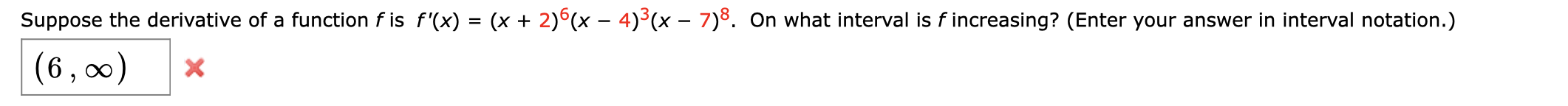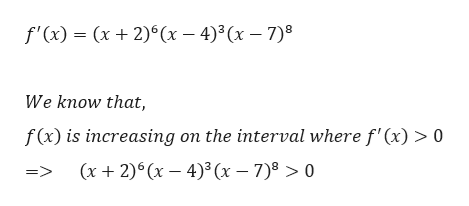# Suppose the derivative of a function f is f'(x) = (x + 2)°(x - 4)5(x - 7)8. On what interval is f increasing? (Enter your answer in interval notation.)(6, 00)X

Question
93 viewshelp_outlineImage TranscriptioncloseSuppose the derivative of a function f is f'(x) = (x + 2)°(x - 4)5(x - 7)8. On what interval is f increasing? (Enter your answer in interval notation.) (6, 00) X fullscreen
check_circle

star
star
star
star
star
1 Rating
Step 1

Given,

...help_outlineImage Transcriptionclosef'(x) x 2)(x 43(x 7) We know that, f(x) is increasing on the interval where f'(x) > 0 (x 2)6 (x 4)(x 7) > 0 => fullscreen

### Want to see the full answer?

See Solution

#### Want to see this answer and more?

Solutions are written by subject experts who are available 24/7. Questions are typically answered within 1 hour.*

See Solution
*Response times may vary by subject and question.
Tagged in

### Derivative